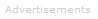# Definition of Volume

What is Volume?

Volume measures size in three dimensions just as length measures size in one dimension and area measures size in two dimensions.

The volume of any cuboid can be calculated by multiplying its length x width x height:

V = L x W x H

where length, width, and height are all at right-angles to one another.

The volumes of other 3D-objects or spaces can be calculated from known formulae. For example, the volume of a sphere is:

V = 43πr3

The SI unit of volume is the cubic meter: m3. Often it is more convenient in chemistry to quote volumes in cubic centimeters: cm3, or cubic decimeters: dm3. The cubic decimeter is the preferred scientific name of the quantity previously referred to as the liter.Search the Dictionary for More Terms